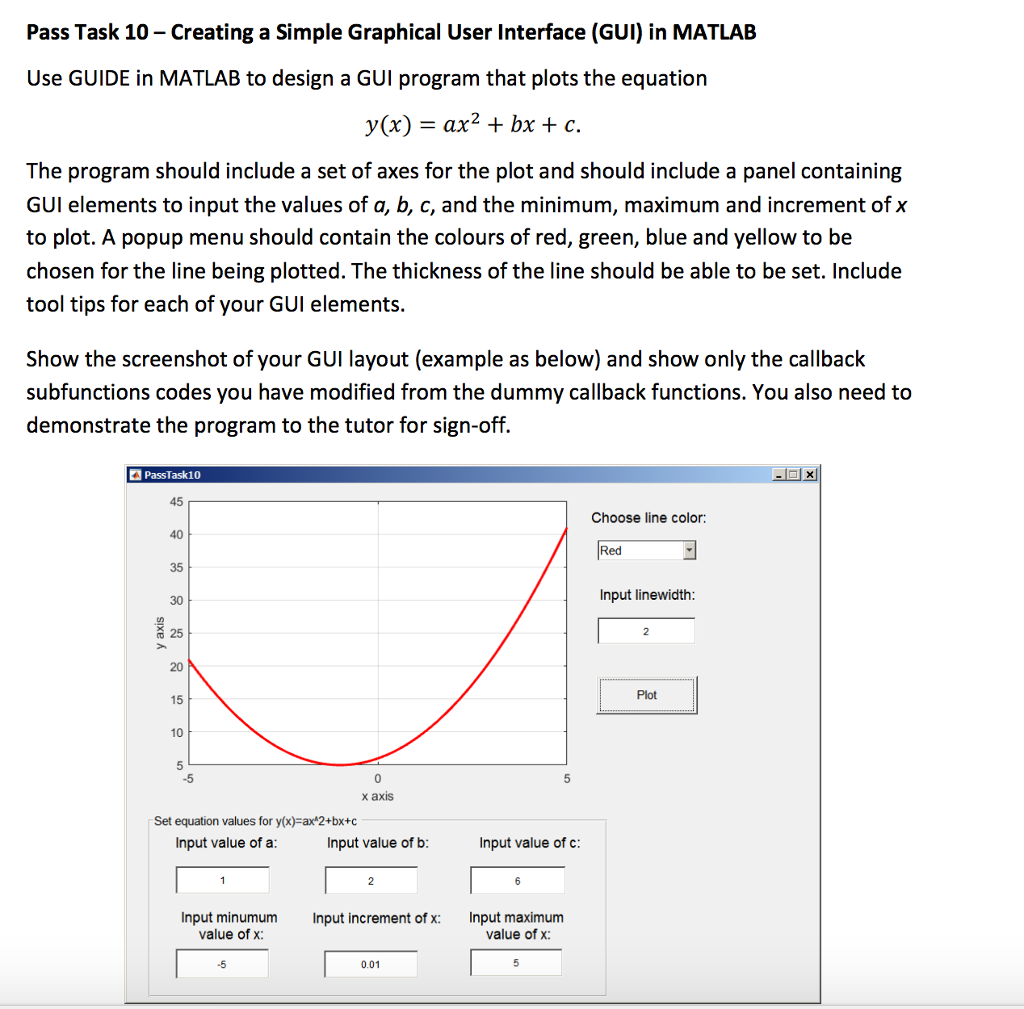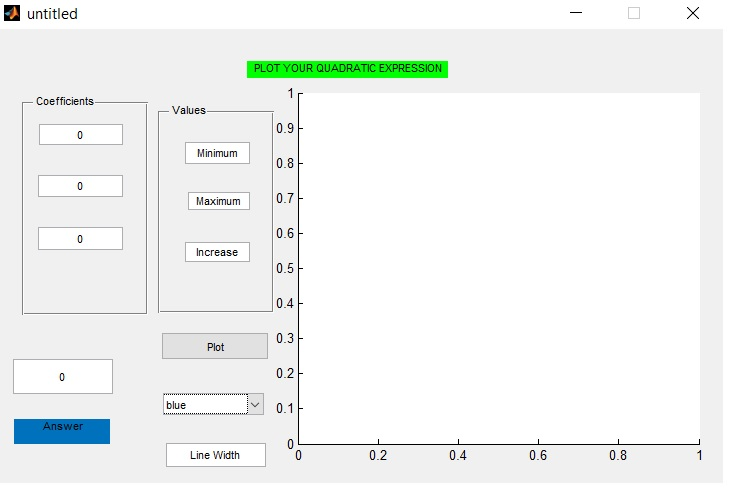# Question & Answer: Creating a Simple Graphical User Interface (GUI) in MATLAB Use GUIDE in MATLAB to design a…..Creating a Simple Graphical User Interface (GUI) in MATLAB Use GUIDE in MATLAB to design a GUI program that plots the equation y(x) = ax^2 + bx + c. The program should include a set of axes for the plot and should include a panel containing GUI elements to input the values of a, b, c, and the minimum, maximum and increment of x to plot. A popup menu should contain the colours of red, green, blue and yellow to be chosen for the line being plotted. The thickness of the line should be able to be set. Include tool tips for each of your GUI elements. Show the screenshot of your GUI layout (example as below) and show only the callback subfunctions codes you have modified from the dummy callback functions. You also need to demonstrate the program to the tutor for sign-off.

Don't use plagiarized sources. Get Your Custom Essay on
Question & Answer: Creating a Simple Graphical User Interface (GUI) in MATLAB Use GUIDE in MATLAB to design a…..
GET AN ESSAY WRITTEN FOR YOU FROM AS LOW AS \$13/PAGE

Using guideThe above image is the layout of the GUI created using guide on MATLAB. It plots ax^2+bx+c where values of a,b, and c are entered in the text box under the panel ‘COEFFICIENTS’ . The panel named ‘Values’ has the range of x .

I have also added a text field to evaluate the value of the equation at any value of X.

Color of plot and line width can be changed using the bottom fields.

Callback function of the button named ‘plot’–>

function pushbutton2_Callback(hObject, eventdata, handles)
% hObject handle to pushbutton2 (see GCBO)
% eventdata reserved – to be defined in a future version of MATLAB
% handles structure with handles and user data (see GUIDATA)
a=str2double(get(handles.edit1,’String’));
b=str2double(get(handles.edit2,’String’));
c=str2double(get(handles.edit3,’String’));
xmin=str2double(get(handles.edit5,’String’));
xmax=str2double(get(handles.edit6,’String’));
xstep=str2double(get(handles.edit7,’String’));